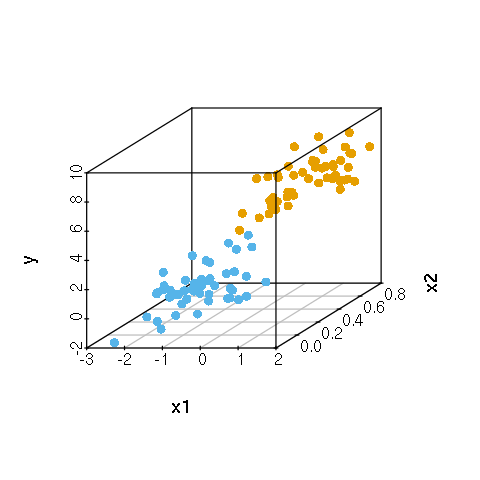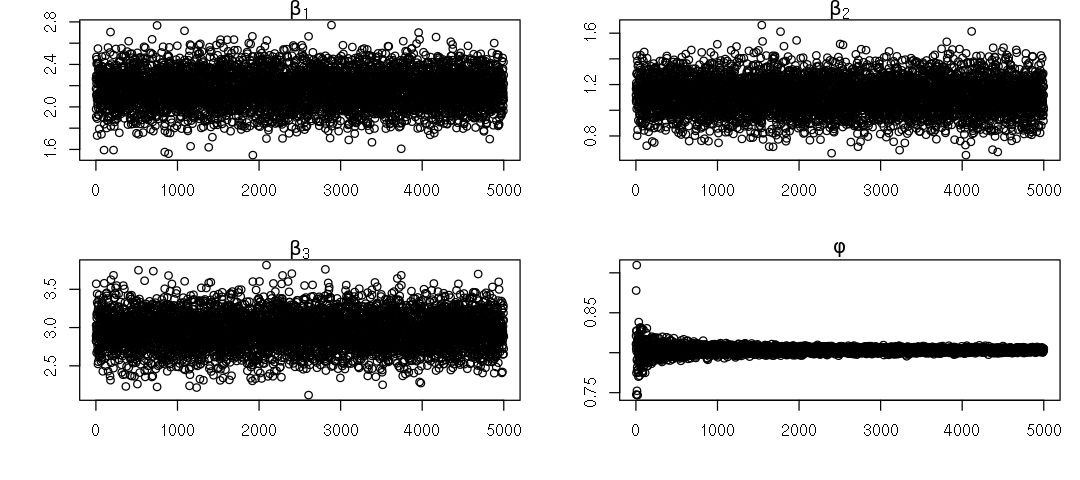# Bayesian Linear Regression¶

Oct. 3, 2018 $\newcommand{\bs}{\boldsymbol}$ $\newcommand{\argmin}{\underset{\bs{#1}}{\text{arg min}}\,}$ $\newcommand{\argmax}{\underset{\bs{#1}}{\text{arg max}}\,}$ $\newcommand{\tr}{^{\top}}$ $\newcommand{\norm}{\left|\left|\,#1\,\right|\right|}$ $\newcommand{\given}{\,|\,}$

## Frequentist Linear Model¶

Let $\bs{Y}$ be a $(n\times 1)$ response vector. Let $\bs{X}$ be the $(n\times p)$ design matrix, where $p-1$ is the number of features. Let $\bs{\beta}$ of dimension $(p\times 1)$ be the vector of regression coefficients. The frequentist version of linear model assumes that

$$\bs{Y} = \bs{X} \bs{\beta} + \bs{\varepsilon},\tag{1}$$

where

$$\bs{Y} \given \bs{X}, \bs{\beta} \sim \mathcal{N}\left(\bs{X}\bs{\beta}, \sigma^2 \bs{I}\right),\tag{2}$$

Equivalently, we have that the $\bs{\varepsilon}$ follows a multivariate normal distribution centered at 0 with uniform variance and mutual independence among the components.

The frequentist obtaines a point estimate for $\bs{\beta}$ by optimizing the $L_2$ loss (or equivalently by maximizing the likelihood).

\begin{aligned} \widehat{\bs{\beta}} &= \argmin{\bs{\beta}} \frac{1}{2}\norm{\bs{Y} - \bs{X}\bs{\beta}}_2 \\ &=\argmax{\bs{\beta}} L(\bs{\beta} \given \bs{X}, \bs{Y}). \end{aligned}

Let $D$ denote the Jacobian. By the multivariate chain rule, we have

\begin{aligned} D \frac{1}{2}\norm{\bs{Y} - \bs{X}\bs{\beta}}_2 &= (\bs{Y}-\bs{X}\bs{\beta})\tr D(\bs{Y}-\bs{X}\bs{\beta}) \\ &= -(\bs{Y}-\bs{X}\bs{\beta})\tr \bs{X} \end{aligned}

The Jacobian is a row vector, so we take its transpose and setting it equal to 0 to obtain

\begin{aligned} \widehat{\bs{\beta}} &= (\bs{X}\tr \bs{X})^{-1} \bs{X}\tr \bs{Y}\\ &\sim \mathcal{N}\left(\bs{\beta}, \sigma^2 (\bs{X}\tr\bs{X})^{-1} \right). \end{aligned} \tag{3}

To do a simple simulation study, we generate 100 bivariate data points where the first variable follows a standard normal distribution and the second follows a binomial distribution with $n=100$ and $p=0.5$. This can be easily achieved in R.

In :
set.seed(3)
n <- 100
X <- cbind(1,rnorm(n),rbinom(n, 1, 0.5))
beta <- c(2, 1, 3)
Y <- X %*% beta + rnorm(n, 0, 1)
print(X[1:5,])

     [,1]       [,2] [,3]
[1,]    1 -0.9619334    1
[2,]    1 -0.2925257    1
[3,]    1  0.2587882    0
[4,]    1 -1.1521319    0
[5,]    1  0.1957828    1


We can compute the estimated coefficient for $\beta$ as follows

In :
beta.MLE <- solve(t(X)%*%X)%*%t(X)%*%Y
print(t(beta.MLE))

         [,1]     [,2]    [,3]
[1,] 2.164229 1.113361 2.96417


A more fancy way is to use the built-in lm function in R.

In :
library(dplyr)
library(data.table)

data <- cbind(X, Y) %>% as.data.table()
names(data) <- c('x0', 'x1', 'x2', 'y')

fit1=lm(y~x1+x2,data=data)

In :
summary(fit1)

Call:
lm(formula = y ~ x1 + x2, data = data)

Residuals:
Min       1Q   Median       3Q      Max
-1.99140 -0.75834  0.04903  0.83442  2.53981

Coefficients:
Estimate Std. Error t value Pr(>|t|)
(Intercept)   2.1642     0.1625   13.32  < 2e-16 ***
x1            1.1134     0.1322    8.42 3.41e-13 ***
x2            2.9642     0.2254   13.15  < 2e-16 ***
---
Signif. codes:  0 ‘***’ 0.001 ‘**’ 0.01 ‘*’ 0.05 ‘.’ 0.1 ‘ ’ 1

Residual standard error: 1.126 on 97 degrees of freedom
Multiple R-squared:  0.7221,	Adjusted R-squared:  0.7164
F-statistic:   126 on 2 and 97 DF,  p-value: < 2.2e-16


We see that the strengh of the linear relationship is very strong. Indeed, the true distribution from which the data is generated is linear! (If we add more noise to the data by increasing the variance of $\bs{\varepsilon}$ without increasing the number of generated data points, the output p-value will increase.)

Just for fun, let's make a scatter plot of the data, where colors represent the value of the binomial random variable 'x2'.

In :
library(scatterplot3d)

colors <- c("#56B4E9", "#E69F00")
colors <- colors[data$x2+1] options(repr.plot.width=4, repr.plot.height=4) scatterplot3d(data[,2:4], pch=16, color=colors)## Bayesian Linear Model¶ The Bayesian linear model makes the same assumption (1) and (2). In addition, we let$\phi = 1/\sigma^2, and assume the following prior distributions \begin{aligned} \bs{\beta} &\sim \mathcal{N}\left(\bs{a}, \phi^{-1} \bs{R}\right) \\ \phi &\sim \text{Gamma}(a_0, b_0) \end{aligned} Substituting in various distributions, the posterior distribution is given by \begin{aligned} p(\bs{\beta}, \phi \given \bs{Y}) &\propto p(\bs{Y} \given \bs{\beta}, \phi) p(\bs{\beta}\given \phi) p(\phi) \\ &\propto \phi^{\frac{n}{2}} \text{exp}\left(-\phi \frac{(\bs{Y}-\bs{X}\bs{\beta})\tr (\bs{Y}-\bs{X}\bs{\beta})}{2}\right) \\ &\quad\quad \times \phi^{\frac{p}{2}} \text{exp}\left(-\phi \frac{(\bs{\beta}-\bs{a})\tr \bs{R}^{-1}(\bs{\beta}-\bs{a})}{2}\right) \\ &\quad\quad \times \phi^{a_0-1}\text{exp}(-\phi b_0). \end{aligned} We are interested in marginal distributions of\beta\given \bs{Y}$and$\phi \given \bs{Y}. Unfortunately, this is a luxury we do not have. Instead, we can find the full conditionals. \begin{aligned} p(\bs{\beta}\given \phi, \bs{Y}) &\propto \text{exp}\left(-\frac{\phi}{2} \left\{(\bs{Y}-\bs{X}\bs{\beta})\tr (\bs{Y}-\bs{X}\bs{\beta})+(\bs{\beta}-\bs{a})\tr \bs{R}^{-1}(\bs{\beta}-\bs{a})\right\} \right) \\ &\propto\text{exp}\left( \bs{\beta}\tr (\bs{X}\tr\bs{X}+\bs{R}^{-1})\bs{\beta} - 2(\bs{Y}\tr \bs{X} + \bs{a}\tr \bs{R}^{-1})\bs{\beta}\right). \quad [\text{expand}] \end{aligned} Comparing this to the kernel of the multivariate normal distribution, we conclude that $$\bs{\beta}\given \phi, \bs{Y} \sim \mathcal{N}\left(\bs{\mu_{\beta}}, \bs{\Sigma_{\beta}}\right),\tag{4}$$ where \begin{aligned} \bs{\Sigma_{\beta}} &= \phi^{-1}\left(\bs{X}\tr \bs{X} + \bs{R}^{-1}\right)^{-1} \\ \bs{\mu_{\beta}} &= \left(\bs{X}\tr \bs{X} + \bs{R}^{-1}\right)^{-1}\left(\bs{R}^{-1}\bs{a}+\bs{X}\tr \bs{Y}\right). \end{aligned}\tag{5} Comparing (5) with (3), we see a lot of similarity. The only difference now is that the posterior full conditional mean is affected by the prior distribution. Similarly, the full conditional distribution of\phiis given by the following. \begin{aligned} p(\phi \given \bs{\beta}, \bs{Y}) &\propto \phi^{\frac{n+p}{2}+a_0-1} \text{exp}\left(-\phi \left\{\frac{1}{2} (\bs{Y}-\bs{X}\bs{\beta})\tr(\bs{Y}-\bs{X}\bs{\beta}) + \frac{1}{2}(\bs{\beta}-\bs{a})\tr(\bs{\beta}-\bs{a}) + b_0\right\}\right). \end{aligned} Immediately we recognize that this is the kernel of a gamma distribution. That is, $$\phi \given \bs{\beta}, \bs{Y} \sim \text{Gamma}\left(\alpha_{\phi}, \beta_{\phi}\right), \tag{6}$$ where \begin{aligned} \alpha_{\phi} &= \frac{n+p}{2} + a_0 \\ \beta_{\phi} &= \frac{1}{2} (\bs{Y}-\bs{X}\bs{\beta})\tr(\bs{Y}-\bs{X}\bs{\beta}) + \frac{1}{2}(\bs{\beta}-\bs{a})\tr(\bs{\beta}-\bs{a}) + b_0. \end{aligned} \tag{7} ## Gibbs sampling¶ The idea of Gibbs sampling is simple, yet the method is extremely powerful. We follow the following prodecure. 1. Initialize the value\phi^{(0)}$. 2. Generate a random sample of$\bs{\beta}^{(1)}$using (4) and (5). 3. Given$\bs{\beta}^{(1)}$, we use (6) and (7) to generate$\phi^{(1)}$. 4. Given$\phi^{(1)}$, we go back to (4) and (5) and generate$\bs{\beta}^{(2)}$. 5. Repeat the above process until we get enough sample points. The Gibbs sampler is an example of a Markov Chain Monte Carlo (MCMC) algorithm that satisfies the following properties. • Each parameter only depends on its previous iteration. Hence it has the Markov property. • The empirical distribution$(\bs{\beta}^{(i)}, \phi^{(i)})$approaches the posterior distribution as$i \to \infty$regardless of the initial value$\phi^{(0)}$. • As the number of sampled points go to infinity, the sample average approaches the posterior mean for each parameter. Let's build our model in R! In : require(mvtnorm)  In : Gibbs.MLR <- function(X, Y, a, R, a0=0, b0=0, iterations=5000, phi=1, plot=TRUE) { # Parameters # ---------- # X = design matrix ------ shape (n, p) # Y = response vector ------ shape (n, 1) # a = prior mean for beta ------ shape (p, 1) # R = prior covariance matrix for beta ------ shape (p, p) # a0, b0 = prior parameters for phi ------ phi~Gamma(a0,b0) # iterations = number of Gibbs iterations # phi = initial value of phi ------- a scalar # Number of training examples n <- dim(X) # Number of covariates p <- dim(X) # Creating placeholder for the parameters beta.MCMC <- matrix('na',nrow=iterations,ncol=p) phi.MCMC <- rep('na',iterations) # Storing various pieces of the matrix products Ri <- solve(R) Ria <- Ri %*% a XtXRi <- solve(t(X) %*% X + Ri) XtY <- t(X) %*% Y # Begin Gibbs sampling! for(i in 1:iterations) { # Generaing the first beta1 using the initialized value beta.sigma <- XtXRi/phi beta.mu <- XtXRi %*% (Ria + XtY) beta <- as.vector(rmvnorm(1, beta.mu, beta.sigma)) # Updating the value of (a0, b0) a0 <- (n + p)/2 + a0 b0 <- (sum((Y - X %*% beta)^2) + (beta - a) %*% Ri %*% (beta - a))/2 + b0 # Getting updated value of phi1 phi <- rgamma(1, a0, b0) # Storing value of beta as a row of the matrix beta.MCMC[i,] <- beta # Storing value of phi phi.MCMC[i] <- phi } if(plot==TRUE) { options(repr.plot.width=9, repr.plot.height=4) par(mfrow=c(2, 2), mar=c(4, 4, 1, 1)) plot(beta.MCMC[,1], ylab="", xlab="", main=bquote(beta)) plot(beta.MCMC[,2], ylab="", xlab="", main=bquote(beta)) plot(beta.MCMC[,3], ylab="", xlab="", main=bquote(beta)) plot(phi.MCMC, ylab="", xlab="", main=bquote(phi)) } return(list("beta" = beta.MCMC, "phi" = phi.MCMC)) }  In : parameters <- Gibbs.MLR(X, Y, a=c(0,0,0), R=diag(rep(10,3)))The Markov chain converges to a stationary distribution easily! Interestingly, we see that the distribution of$\phi$has a high variance initially. So in order to obtain a point estimate, we can truncate the initial portions of the chain. In : # Finding the posterior mean of beta and phi parameters$beta[2500:5000, 1] %>% as.numeric() %>% mean()
parameters$beta[2500:5000, 2] %>% as.numeric() %>% mean() parameters$beta[2500:5000, 3] %>% as.numeric() %>% mean()
parameters$phi[2500:5000] %>% as.numeric() %>% mean()  2.16551830157711 1.11273106085075 2.95906440725969 0.803247806992739 Recall that using frequentist MLE we obtained$\widehat{\bs{\beta}} = (2.164229, 1.113361, 2.96417)\$. This is indeed extremely close to the frequentist estimation.